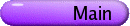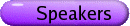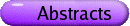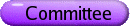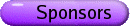Harmonic Analysis
Org: Izabella Laba (UBC) and Malabika Pramanik (Caltech; UBC)
[PDF]

CHRISTINE CARRACINO, Richard Stockton College of NJ, Pomona, NJ 08240
Estimates for the Szegö kernel and the [`()]b equation
[PDF]

We can consider the Szegö kernel S(z,z) on the boundary of model domains W = {(z1,z2) Î C2 | - Áz2 > b(Âz1)}. If b is convex, the only singularities of S(z,z) are on the diagonal z=z. When the function b is a certain non-convex function, we show that near certain points, there are singularities off the diagonal.

We will then discuss some recent work on a related problem, the [`()]b problem for the model domain considered above.

This is joint work with Jennifer Halfpap.

JIM COLLIANDER, University of Toronto
Strichartz refinements and concentration in NLS
[PDF]

Refinements of the Fourier restriction estimate have been used by Bourgain and others to prove mass concentration occurs in finite time blowup solutions of mass critical nonlinear Schrodinger equations. I'll revisit this argument and remark on certain quantifications and extensions.

GALIA DAFNI, Department of Mathematics and Statistics, Concordia University, 1455 de Maisonneuve Blvd. West, Montreal, Quebec, H3G 1M8, Canada
Classes of measures generated by capacities
[PDF]

For b < 1, the fractional Carleson condition for a measure m on the upper half-space Rn+1+:

 m æè T(B) öø £ C |B|b
for all balls B in Rn, where |B| is Lebesgue measure and T(B) is the tent over B, is not equivalent to the same condition for open sets. In joint work with Georgi Karadzhov and Jie Xiao, we show that these Carleson-type conditions (involving balls) are equivalent to conditions bounding the measure of the tent T(O) over an open set O by a function of its capacity. The capacities used include Riesz, Bessel, Besov and Hausdorff capacities. These conditions are analogous to conditions introduced by Maz'ya for measures on Rn.

BURAK ERDOGAN, University of Illinois at Urbana-Champaign
Strichartz estimates for Schrodinger equations with large magnetic potentials
[PDF]

We show that the time evolution of the magnetic Schrodinger operator

 H = -D+ i(A·Ñ+ Ñ·A) + V
in R3 satisfies global Strichartz and smoothing estimates under suitable smoothness and decay assumptions on A and V but without any smallness assumptions. We require that zero energy is neither an eigenvalue nor a resonance.

This is a joint work with M. Goldberg and W. Schlag.

RALUCA FELEA, Rochester Institute of Technology
Composition calculus in inverse scattering
[PDF]

Fourier integral operators (FIOs) with singularities are used in inverse scattering theory. In such problems, artifacts can appear when inversion is attempted and one would like to understand precisely and eliminate them as far as possible. The operators considered here arise in linearized seismic imaging and synthetic aperture radar. The singularities which appear in this problems are folds, submersion with folds and cross caps. One would like to understand the composition of such operators since in general, the composition of two FIOs is not a FIO. We will establish a composition calculus for FIOs associated to folding canonical relations, working away from the fold points.

MICHAEL GREENBLATT, University at Buffalo
A resolution of singularities and some applications
[PDF]

A geometric resolution of singularities algorithm for real-analytic functions is described. This method is elementary in its statement and proof, extensively using explicit coordinate systems. Each coordinate change used in the resolution procedure is one-to-one on its domain, and is of one of a few explicit canonical forms. Applications to classical analysis are given, including a theorem regarding the existence of critical integrability exponents.

ALLAN GREENLEAF, University of Rochester, Department of Mathematics, Rochester, NY 14627, USA
Counterexamples to Calderón's problem as the mathematics of invisibility
[PDF]

We show how earlier work on counterexamples for the Calderón problem (i.e., whether a conductivity function or tensor s(x) on a domain W is determined by the boundary values of the solutions to Ñ·(sÑ)u = 0) can be extended to obtain rigorous results concerning invisibility (or "cloaking") for solutions of the Helmholtz and Maxwell equations.

This is joint work with Yaroslav Kurylev, Matti Lassas and Gunther Uhlmann.

KATHRYN HARE, Department of Pure Mathematics, University of Waterloo, 200 University Ave. W., Waterloo, Ontario, N2L 3G1
Directional maximal operators with smooth densities
[PDF]

We investigate the mapping properties of directional maximal operators on the plane with smooth densities. If vectors of all lengths in a given set of directions are taken in defining the maximal operator, then the boundedness of the operator depends on the order of the stationary points of the density function. In contrast, if the set of directions is limited to sums of diadic directions, and the density function has only finitely many stationary points, each of finite order, the maximal operator is bounded on all Lp for p > 1.

This is joint work with M. Roginskaya.

ALEX IOSEVICH, Missouri-Columbia

Discrete Schrodinger Operators with Slowly Oscilating Potentials
[PDF]

We investigate asyptotic behavior of the generalized eigenfunctions of discrete Schrodinger operator with potentials b(n) Î lp(Z), 1 < p < 2. Our approach is based on the work of Christ and Kiselev in the continuous case. The main new step is a development of discrete version of multilinear operator analysis and maximal function estimate.

Joint work with A. Kiselev.

SUNG EUN KIM, University of Rochester, Rochester, NY
Calderon's inverse problem for piecewise linear conductivities
[PDF]

We will show the global uniqueness for the Calderon's inverse problem for a piecewise linear, or more generally piecewise smooth across polyhedra, conductivity in R3 by using the geometry of Faddeev Green's function.

MICHAEL LACEY, Geogia Tech
On the Small Ball Problem
[PDF]

We consider Haar functions in the unit cube in three dimensions, normalized in L¥. The question at hand is a `non-trivial' lower bound on the L¥ norm of the sum

 å |R|=2-n aR hR (x).
The key point of the sum is that is formed over rectangles of a fixed volume-this is the `Hyperbolic' assumption. We prove that for some h > 0, we have the estimate
 || å |R|=2-n aR hR (x) ||¥ > cn-1+h2-n å |R|=2-n |aR|
(h = 0 is the `trivial' estimate). In a prior result of J. Beck, a famous and famously difficult result, established a logarithmic gain over the trivial estimate. We simplify and extend Beck's argument to prove this result.

Joint work with Dmitry Bilyk.

NEIL LYALL, University of Georgia
On a Theorem of Sárközy and Furstenberg
[PDF]

A theorem of Sárközy and Furstenberg states that if A Í Z with positive upper Banach density, then the set of quadratic return times {d | AÇ(A+d2) ¹ Æ} is non-empty. Using Fourier analysis we give a new proof of the fact that the set of all quadratic return times is syndetic and obtain uniform lower bounds for the density of these return times.

This is joint work with Ákos Magyar.

AKOS MAGYAR, University of Georgia
Maximal operators associated to discrete subgroups of nilpotent Lie groups
[PDF]

A maximal theorem is proved for averages taken over suitable discrete sub-varieties of nilpotent Lie groups.

Imaging Obstacles In Inhomogeneous Media
[PDF]

We show that an obstacle inside a known inhomogeneous medium can be determined from the scattering amplitude at one frequency. Moreover, we show that if the scattering operators for two obstacles are comparable at one non-resonant frequency, then the obstacles must coincide. The proof gives a new reconstruction procedure; it is based on an extension of the factorization method, which I'll review.

This is joint work with Lassi Paivarinta and Ari Teirila.

ALEXANDER NAGEL, Department of Mathematics, University of Wisconsin, 480 Lincoln Drive, Madison, WI 53706, USA
Bergman and Szego kernels on tubular domains near points of infinite type
[PDF]

In joint work with Jennifer Halfpap and Stephen Wainger, we obtain estimates for the Bergman and Szegö kernels in domains of the form

 W = ìí î (z1, z2) Î C2 êê Á[z2] > b (Â[z1]) üý þ
where b Î C¥ (R) is convex and even, with b"(r) > 0 for |r| > 0 and b(n)(0) = 0 for all non-negative integers n. For example, if ba(r) = exp(-|r|-a) for |r| small, we show that the Bergman and Szegö kernels have singularities away from the diagonal of the boundary of W if and only if a ³ 1.

The (d,k) Kakeya problem
[PDF]

A (d,k) set is a subset of Rd containing a translate of every k-dimensional plane. We use mixed-norm estimates for the x-ray transform to obtain improved dimension estimates for (d,k) sets.

JILL PIPHER, Brown University
Square function variants adapted to boundary value problems
[PDF]

A variant of the square function has applications to boundary value problems and to characterizing A¥ weights.

ERIC SAWYER, McMaster University, Hamilton, Ontario
Interpolating sequences for the Dirichlet space
[PDF]

In the 1950's Buck raised the question of whether or not there exists an infinite subset Z = {zj}j=1¥ of D that is interpolating for H¥(D). In 1958 Carleson gave an affirmative answer and characterized all such interpolating sequences in the disk. In 1961 Shapiro and Shields demonstrated the equivalence of this problem with certain Hilbert space analogues involving l2(m) where m = åj kzj(zj)-1dzj and kz is the reproducing kernel. Suppose that Z = {zj}j=1¥ Ì D is separated and let R be the restriction map Rf = {f(zj)}j=1¥. Then the results of Carleson and Shapiro and Shields show the following four conditions are equivalent: R maps H¥(D) onto l¥(Z); R maps H2(D) onto l2(m); R maps H2(D) into l2(m); m( T(I) ) £ C|I| for all arcs I Ì T. In 1995 Marshall and Sundberg extended this theorem to the Dirichlet space D(D) and its multiplier algebra MD(D), but without the second condition. In fact, as observed by Bishop in 1995, even when the measure m is finite, R maps D(D) onto l2(m) for sequences Z more general than those for which R maps D(D) into l2(m). We give two results toward resolving the open question of when R maps D(D) onto l2(m): the first is a geometric characterization of such Z in the case m is a finite measure, and the second shows there are such Z with m an infinite measure, thus answering a question of Bishop.

Fourier restriction theorems for curves
[PDF]

We shall report recent joint work with Jong-Guk Bak and Daniel Oberlin on Fourier restriction for curves in Rd and related oscillatory integral operators.

ROMAN SHTERENBERG, University of Wisconsin-Madison, Math. Dept., 480 Lincoln Dr., Madison, WI 53706-1388, USA
Blow up and regularity for Burgers equation with fractional dissipation
[PDF]

We present a comprehensive study of the existence, blow up and regularity properties of solutions of the Burgers equation with fractional dissipation. We prove existence of the finite time blow up for the power of Laplacian a < 1/2, and global existence as well as analyticity of solution for a ³ 1/2. We also discuss solutions with very rough initial data.

GIGLIOLA STAFILLANI, MIT, Room 2-246, 77 Massachusetts Ave., Cambridge, MA 02139
On the L2 critical NLS
[PDF]

In this talk I will give an overview of the global well-posedness results for the L2 critical NLS below the H1 norm. The recent result of Tao-Visan-Zhang proves global well-posedness in L2 for radial data in higher dimensions d ³ 3. This problem is still open in lower dimensions and for nonradial data. In this talk I will present some recent results of global well-posedness that I obtained with De Silva, Pavlovic and Tzirakis for any data in Hsd for a certain 0 < sd < 1.

PAUL TAYLOR, Shippensburg University, 1871 Old Main Dr., Shippensburg, PA 17011, USA
Bochner-Riesz Means With Respect to a 2 by 2 Cylinder
[PDF]

We present estimates for Bochner-Riesz means with respect to the R2 ×R2 cylinder variant { x, max{x12 + x22, x32 + x42} = 1 }. The operator Sl to be studied is given by

 ^ Slf (x) = æè 1 - max ìí î Ö x12 + x22 , Ö x32 + x42 üý þ öø l+ .

ERIN TERWILLEGER, University of Connecticut
The Wiener-Wintner Theorem for the Hilbert Transform
[PDF]

Our main result is an oscillation inequality which is an extension of Carleson's Theorem on Fourier series. As a consequence one obtains the following extension of the Wiener-Wintner Theorem on ergodic averages: for all measure preserving flows (X,m,Tt) and f Î Lp(X,m), there is a set Xf Ì X of probability one, so that for all x Î Xf we have

 lim s¯ 0 óõ s < |t| < 1/s eiqt f(Tt x) dt t exists for all q.

This work is joint with Michael Lacey.